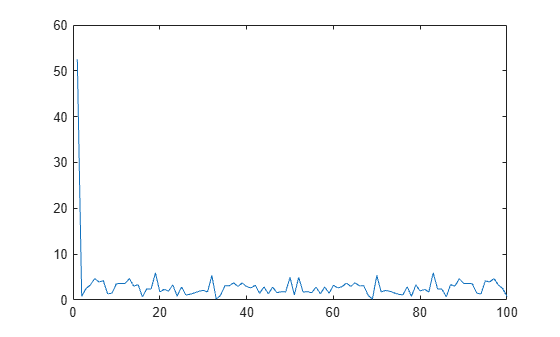This example shows how to create two different memory maps, and then read from each of the maps using the appropriate syntax. Then, it shows how to modify map properties and analyze your data.

You can read the contents of a file that you mapped to memory using the same MATLAB® commands you use to read variables from the MATLAB workspace. By accessing the `Data` property of the memory map, the contents of the mapped file appear as an array in the currently active workspace. To read the data you want from the file, simply index into the array. For better performance, copy the `Data` field to a variable, and then read the mapped file using this variable:

`dataRef = m.Data; `

`for k = 1 : N `

` y(k) = dataRef(k);`

`end `

By contrast, reading directly from the `memmapfile` object is slower:

`for k = 1 : N `

` y(k) = m.Data(k);`

`end `

### Read from Memory Map as Numeric Array

First, create a sample data file named `records.dat` that contains a 5000-by-1 matrix of double-precision floating-point numbers.

```rng('default') randData = rand([5000,1]); fileID = fopen('records.dat','w'); fwrite(fileID,randData,'double'); fclose(fileID);```

Map 100 double-precision floating-point numbers from the file to memory, and then read a portion of the mapped data. Create the memory map, `m`. Specify an `Offset` value of 1024 to begin the map 1024 bytes from the start of the file. Specify a `Repeat` value of 100 to map 100 values.

```m = memmapfile('records.dat','Format','double', ... 'Offset',1024,'Repeat',100);```

Copy the `Data` property to a variable, `d`. Then, show the format of `d`.

```d = m.Data; whos d```
``` Name Size Bytes Class Attributes d 100x1 800 double ```

The mapped data is an 800-byte array because there are 100 `double` values, each requiring 8 bytes.

Read a selected set of numbers from the file by indexing into the vector, `d`.

`d(15:20)`
```ans = 6×1 0.3510 0.5132 0.4018 0.0760 0.2399 0.1233 ```

### Read from Memory Map as Nonscalar Structure

Map portions of data in the file, `records.dat`, as a sequence of multiple data types.

Call the `memmapfile` function to create a memory map, `m`.

``` m = memmapfile('records.dat', ... 'Format', { ... 'uint16' [5 8] 'x'; ... 'double' [4 5] 'y' });```

The `Format` parameter tells `memmapfile` to treat the first 80 bytes of the file as a 5-by-8 matrix of `uint16` values, and the 160 bytes after that as a 4-by-5 matrix of `double` values. This pattern repeats until the end of the file is reached.

Copy the `Data` property to a variable, `d`.

`d = m.Data`
```d=166×1 struct array with fields: x y ```

`d` is a 166-element structure array with two fields. `d` is a nonscalar structure array because the file is mapped as a repeating sequence of multiple data types.

Examine one structure in the array to show the format of each field.

`d(3)`
```ans = struct with fields: x: [5x8 uint16] y: [4x5 double] ```

Read the `x` field of that structure from the file.

`d(3).x`
```ans = 5x8 uint16 matrix 62645 30353 12492 16358 58958 9377 48754 16323 14152 21370 16352 21042 61010 33482 16321 22042 2657 16336 37389 35249 45699 16353 47136 59002 16360 41668 9638 33351 16366 3344 58286 31491 5368 55234 24278 16364 55768 7216 7184 16336 ```

MATLAB formats the block of data as a 5-by-8 matrix of `uint16` values, as specified by the `Format` property.

Read the `y` field of that structure from the file.

`d(3).y`
```ans = 4×5 0.8407 0.9293 0.6160 0.5853 0.7572 0.2543 0.3500 0.4733 0.5497 0.7537 0.8143 0.1966 0.3517 0.9172 0.3804 0.2435 0.2511 0.8308 0.2858 0.5678 ```

MATLAB formats the block of data as a 4-by-5 matrix of `double` values.

### Modify Map Properties and Analyze Data

This part of the example shows how to plot the Fourier transform of data read from a file via a memory map. It then modifies several properties of the existing map, reads from a different part of the data file, and plots a histogram from that data.

Create a sample file named `double.dat`.

```randData = rand([5000,1]); fileID = fopen('double.dat','w'); fwrite(fileID,randData,'double'); fclose(fileID);```

Create a `memmapfile object` of 1,000 elements of type `double`, starting at the 1025th byte.

```m = memmapfile('double.dat','Offset',1024, ... 'Format','double','Repeat',1000);```

Copy the `Data` property to a variable, `k`. Then, get data associated with the map and plot the FFT of the first 100 values of the map.

```k = m.Data; plot(abs(fft(k(1:100))))```This is the first time that data is referenced and is when the actual mapping of the file to the MATLAB address space takes place.

Change the map properties, but continue using the same file. Whenever you change the value of a memory map property, MATLAB remaps the file to memory.

```m.Offset = 4096; m.Format = 'single'; m.Repeat = 800;```

`m` is now a `memmapfile` object of 800 elements of type `single`. The map now begins at the 4096th byte in the file, `records.dat`.

Read from the portion of the file that begins at the 4096th byte, and calculate the maximum value of the data. This command maps a new region and unmaps the previous region.

`X = max(m.Data)`
```X = single 3.2278e+38 ```